# C Program to Find Maximum Element in Array

In this tutorial you will learn about the C Program to Find Maximum Element in Array and its application with practical example.

## C Program to Find Maximum Element in Array

In this tutorial, we will learn to create a C program that will find the maximum Element in Array using C programming.

## Prerequisites

Before starting with this tutorial we assume that you are best aware of the following C programming topics:

• Operators in C Programming.
• Basic Input and Output function in C Programming.
• Basic C programming.
• For loop in C Programming.
• Creating and Using the user-defined function in C programming.

## The Maximum value of array:-

As we all know array is a collection of similar data type elements. In an array, only one variable is declared which can store multiple values. The index value of the array start’s from “0” if the array is having size 5 i.e. a = { 1, 2, 3, 4, 5 } values can be stored in that array. Now the a = 1 , a = 2, a = 3, a = 4, a = 5. On the array, we can do many operations with the help of the c language.

We will find the maximum size element with the help of conditional statements.

## Program:-

To find the Maximum value element from the array

## Output:-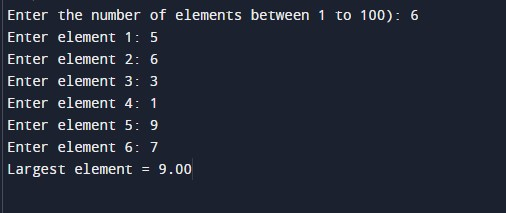In the above program, we have first initialized the required variable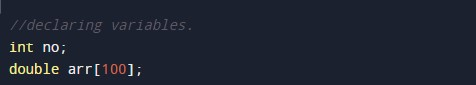• arr = it will hold the elements in an array.
• i = it will hold the integer value to control the array.
• no= it will hold the size of the array.

Printing output of the program to the user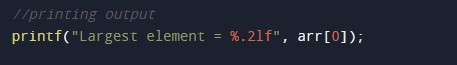Input Size.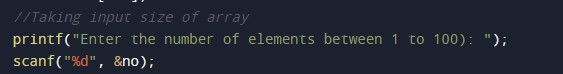Input elements of the array.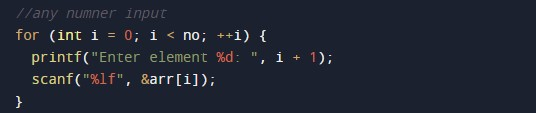Calculating the maximum value.In this tutorial we have learn about the C Program to Find Maximum Element in Array and its application with practical example. I hope you will like this tutorial.